1. /
2. CBSE
3. /
4. Class 08
5. /
6. Mathematics
7. /
8. NCERT Solutions for Class...

# NCERT Solutions for Class 8 Maths Exercise 10.2### myCBSEguide App

Download the app to get CBSE Sample Papers 2023-24, NCERT Solutions (Revised), Most Important Questions, Previous Year Question Bank, Mock Tests, and Detailed Notes.

NCERT solutions for Class 8 Maths Visualising Solid Shapes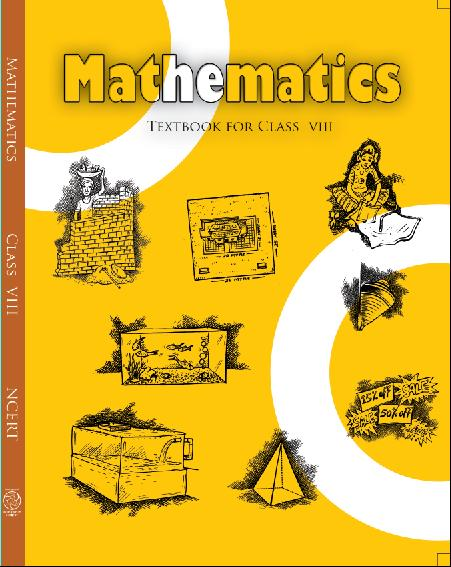## NCERT Solutions for Class 8 Maths Visualising Solid Shapes

Class –VIII Mathematics (Ex. 10.2)

NCERT SOLUTION

1. Can a polygon have for its faces:

(i) 3 triangles

(ii) 4 triangles

(iii) a square and four triangles

Ans. (i) No, a polyhedron cannot have 3 triangles for its faces.

(ii) Yes, a polyhedron can have four triangles which is known as pyramid on triangular base.

(iii) Yes, a polyhedron has its faces a square and four triangles which makes a pyramid on square base.

###### 2. Is it possible to have a polyhedron with any given number of faces? (Hint: Think of a pyramid)

Ans. It is possible, only if the number of faces are greater than or equal to 4.

NCERT Solutions for Class 8 Maths Exercise 10.2

###### 3. Which are prisms among the following: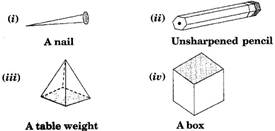Ans. Figure (ii) unsharpened pencil and figure (iv) a box are prisms.

NCERT Solutions for Class 8 Maths Exercise 10.2

###### 4. (i) How are prisms and cylinders alike?

(ii) How are pyramids and cones alike?

Ans. (i) A prism becomes a cylinder as the number of sides of its base becomes larger and larger.

(ii) A pyramid becomes a cone as the number of sides of its base becomes larger and larger.

###### 5. Is a square prism same as a cube? Explain.

Ans. No, it can be a cuboid also.

NCERT Solutions for Class 8 Maths Exercise 10.2

###### 6. Verify Euler’s formula for these solids.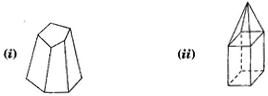Ans. (i) Here, figure (i) contains 7 faces, 10 vertices and 15 edges.

Using Eucler’s formula, we see

F + V – E = 2

Putting F = 7, V = 10 and E = 15,

F + V – E = 2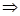7 + 10 – 5 = 217 – 15 = 22 = 2L.H.S. = R.H.S.

(ii)  Here, figure (ii) contains 9 faces, 9 vertices and 16 edges.

Using Eucler’s formula, we see

F + V – E = 2

F + V – E = 29 + 9 – 16 = 218 – 16 = 22 = 2L.H.S. = R.H.S.

Hence verified Eucler’s formula.

NCERT Solutions for Class 8 Maths Exercise 10.2

###### 7. Using Euler’s formula, find the unknown:
 Faces ? 5 20 Vertices 6 ? 12 Edges 12 9 ?

Ans. In first column, F = ?, V = 6 and

E = 12

Using Eucler’s formula, we see

F + V – E = 2

F + V – E = 2F + 6 – 12 = 2F – 6 = 2F = 2 + 6 = 8

Hence there are 8 faces.

In second column, F = 5, V = ? and E = 9

Using Eucler’s formula, we see

F + V – E = 2

F + V – E = 25 + V – 9 = 2V – 4 = 2V = 2 + 4 = 6

Hence there are 6 vertices.

In third column, F = 20, V = 12 and E = ?

Using Eucler’s formula, we see

F + V – E = 2

F + V – E = 220 + 12 – E = 232 – E = 2E = 32 – 2 = 30

Hence there are 30 edges.

NCERT Solutions for Class 8 Maths Exercise 10.2

###### 8. Can a polyhedron have 10 faces, 20 edges and 15 vertices?

Ans. If F = 10, V = 15 and E = 20.

Then, we know Using Eucler’s formula,

F + V – E = 2

L.H.S. = F + V – E

= 10 + 15 – 20

= 25 – 20

= 5

R.H.S.  = 2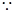L.H.S.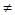R.H.S.

Therefore, it does not follow Eucler’s formula.

So polyhedron cannot have 10 faces, 20 edges and 15 vertices.

## NCERT Solutions for Class 8 Maths Exercise 10.2

NCERT Solutions Class 8 Mathematics PDF (Download) Free from myCBSEguide app and myCBSEguide website. Ncert solution class 8 Mathematics includes text book solutions from Class 8 Maths Book . NCERT Solutions for CBSE Class 8 Maths have total 16 chapters. 8 Maths NCERT Solutions in PDF for free Download on our website. Ncert class 8 solutions PDF and Maths ncert class 8 PDF solutions with latest modifications and as per the latest CBSE syllabus are only available in myCBSEguide.

## CBSE app for Class 8

To download NCERT Solutions for class 8 Social Science, Computer Science, Home Science,Hindi ,English, Maths Science do check myCBSEguide app or website. myCBSEguide provides sample papers with solution, test papers for chapter-wise practice, NCERT solutions, NCERT Exemplar solutions, quick revision notes for ready reference, CBSE guess papers and CBSE important question papers. Sample Paper all are made available through the best app for CBSE students and myCBSEguide website.Test Generator

Create question paper PDF and online tests with your own name & logo in minutes.myCBSEguide

Question Bank, Mock Tests, Exam Papers, NCERT Solutions, Sample Papers, Notes